## 3.2.3 Calling of the derived modules

The derived modules are constructed using COLIB2 for the case where there is only one single block of a given type. There are consequently 3 derived modules: COLIBT , COLIBP  and COLIBH  corresponding to the case of a single tetrahedron, pentahedron or hexahedron.

The data is of the same type as above, but is simpler to enter because some data is implicitly known.

There are two options to assign reference numbers: the one indicates that all these numbers will be set to 0, and the other allows a distinct number to be assigned to each item created.

### COLIBT: tetrahedral case

• dimension array M (in the blank common)
• declare the integer arrays IDECAR(6) and NBPARE(6)
• declare the real arrays XYZ(3,4) and XYZINT(3,LINT) where LINT is the total number of control points input by the user in the 6 coarse edges
• call INITI
• call the module

CALL COLIBT (M,NFNOPO,NINOPO,XYZ,IDECAR,NBPARE,XYZINT,
. IREF,NDSDE)

where

• M is the super array,
• NF(NI)NOPO is the support number of O.D.S. NOPO and its level,
• XYZ(3,4) are the coordinates of the 4 coarse vertices
• IDECAR(I) is the splitting code of the edge: 0 for a straight edge split automatically into segments of the same length; 1 if we input the intermediate points (see XYZINT)
• NBPARE(I) is the number of control points to create on the edge (end-points excluded)
• XYZINT(3,.) are the coordinates of the control points of the edges input by the user. For each edge I for which NDECAR(I) = 1, we enter these values as follows: XYZINT(J,I) is coordinate J of point I, where I is the global number of the point; we first count the points (end-points excluded) of the first edge thus described, followed by those of the second, etc.
• IREF is the option for assigning the references: 0 : all references are set to 0; 1 : each item is differentiated: the vertices from 1 to 4, the edges (and their control points) from 5 to 10, the faces (and the points created on them) from 11 to 14.
• NDSDE is the sub-domain number (of all the sub-elements created)

Remark:

• To change one or several reference numbers (IREF=1), use module MODNOP ( MODNXX) or keyword NUME of APNOP3 after the introduction of the mesh (keyword INTR of APNOP3).

### COLIBP: pentahedral case

• dimension array M (in the blank common)
• declare the integer arrays IDECAR(9) and NBPARE(9)
• declare the real arrays XYZ(3,6) and XYZINT(3,LINT) where LINT is the total number of control points on the 9 coarse edges input by the user
• call INITI
• call the module

CALL COLIBP (M,NFNOPO,NINOPO,XYZ,IDECAR,NBPARE,XYZINT,
. IREF,NDSDE)

where

• M is the super array,
• NF(NI)NOPO is the support number of O.D.S. NOPO and its level,
• XYZ(3,6) are the coordinates of the 6 coarse vertices
• IDECAR(I) is the splitting code of edge I: 0 for a straight edge split automatically into segments of the same length; 1 if we input the intermediate points (see XYZINT)
• NBPARE(I) is the number of control points to create on the edge (end-points excluded)
• XYZINT(3,.) are the coordinates of the control points of the edges input by the user. For each edge I for which NDECAR(I) = 1, we enter these values as follows: XYZINT(J,I) is coordinate J of point I, where I is the global number of the point; we first count the points (end-points excluded) of the first edge thus described, followed by those of the second, etc.
• IREF is the option for assigning the references: 0 : all references are set to 0; 1 : each item is differentiated: the vertices from 1 to 6, the edges (and their control points) from 7 to 15, the faces (and the points created on them) from 16 to 20.
• NDSDE is the sub-domain number (of all the sub-elements created)

Remark:

• To change one or several reference numbers (IREF=1), use module MODNOP ( MODNXX) or keyword NUME of APNOP3 after the introduction of the mesh (keyword INTR of APNOP3).

### COLIBH: hexahedral case

• dimension array M (in the blank common)
• declare the integer arrays IDECAR(12) and NBPARE(12)
• declare the real arrays XYZ(3,8) and XYZINT(3,LINT) where LINT is the total number of control points input by the user on the 12 coarse edges
• call INITI
• call the module

CALL COLIBH (M,NFNOPO,NINOPO,XYZ,IDECAR,NBPARE,XYZINT,
. IREF,NDSDE)

where

• M is the super array,
• NF(NI)NOPO is the support number of O.D.S. NOPO and its level,
• XYZ(3,8) are the coordinates of the 8 coarse vertices
• IDECAR(I) is the splitting code of edge I: 0 for a straight edge split automatically into segments of the same length; 1 if we input the intermediate points (see XYZINT)
• NBPARE(I) is the number of control points to create on the edge (end-points excluded)
• XYZINT(3,.) are the coordinates of the control points of the edges input by the user. For each edge I for which NDECAR(I) = 1, we enter these values as follows: XYZINT(J,I) is coordinate J of point I, where I is the global number of the point; we first count the points (end-points excluded) of the first edge thus described, followed by those of the second, etc.
• IREF is the option for assigning the references: 0 : all references are set to 0; 1 : each item is differentiated: the vertices from 1 to 8, the edges (and their control points) from 9 to 20, the faces (and the points created on them) from 21 to 26.
• NDSDE is the sub-domain number (of all the sub-elements created)

Remark:

• To change one or several reference numbers (IREF=1), use module MODNOP ( MODNXX) or keyword NUME of APNOP3 after the introduction of the mesh (keyword INTR of APNOP3).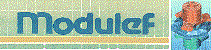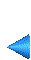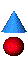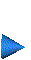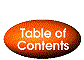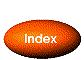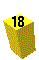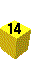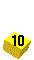Next: 3.2.4 Module COLIBR Up: 3.2 Multi-block method Prev: 3.2.2 Calling of module COLIB2 Index Contents